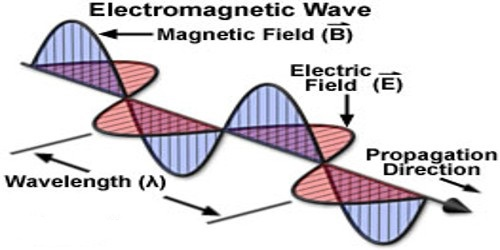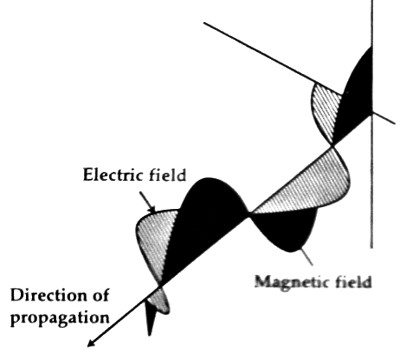# Electromagnetic Theory of Wave

Electromagnetic theory of Wave: In 1845 Faraday discovered that plane of polarization turns due to the influence of strong magnetic field. This effect is known as Faraday’s effect. After the discovery of Faraday’s effect scientists assumed first that there was a close relationship between light and magnetism. According to Faraday’s law of electromagnetic induction electric field is produced the change of magnetic field. So it can be said that light is a form of electromagnetic radiation. Two fields are associated with this radiation. One is the variable electric field and the other one is the variable magnetic field. Hence, it is not unlikely that there s a close relationship between electricity and magnetism with light.

James Clerk Maxwell in 1864, from the result of displacement current in a dielectric medium, proposed that varying electric field also produces magnetic field [Figure]. So, varying electric field (E) and magnetic field (B) creates a type of disturbance in a vacuum.Fig: Electromagnetic theory of Wave

This disturbance has the characteristic of waves. The wave nature of this disturbance is called an electromagnetic wave. Maxwell came to the conclusion that electric field (E) and magnetic field (B) of the electromagnetic waves mutually perpendicular to each other in the same plane and energy of the wave transmitted normal to the plane. The electromagnetic waves travel through a vacuum with velocity,

c = 1/√(ϵ0μ0) … … … (1)

Here ϵ0 is the permittivity of vacuum and its value,

ϵ0 = [1 / (4π x 9 x 109)] C2N-1m-2

and μ0 is the permeability of vacuum whose value, μ0 = 4π x 10-7 NA-2.

If the values of ϵ0 and μ0 are inserted in equation (1), we get the value of c as 3 x 108 ms-1.

That means electromagnetic waves travel in vacuum at the speed of light. So, light waves and electromagnetic waves are identical, the only difference is in their wavelengths. Maxwell also proved that this wave is a transverse wave. In short, it can be said, electric and magnetic vibrations, moving at the speed of light in vacuum, in which electric and magnetic fields are perpendicular to each other and both of them are normal to the direction of propagation of the wave is called an electromagnetic wave.0